# Isaac Newton's Second Law of Motion

The Second Law is concerned with relating acceleration to mass and net force.

Newton's second law of motion explains how an object will change velocity if it is pushed or pulled upon.

Firstly, this law states that if you do place a force on an object, it will accelerate (change its velocity), and it will change its velocity in the direction of the force. So, a force aimed in a positive direction will create a positive change in velocity (a positive acceleration). And a force aimed in a negative direction will create a negative change in velocity (a negative acceleration).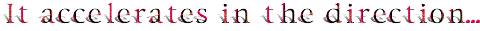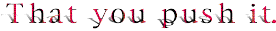Secondly, this acceleration is directly proportional to the force. For example, if you are pushing on an object, causing it to accelerate, and then you push, say, three times harder, the acceleration will be three times greater.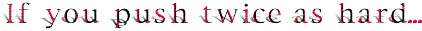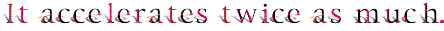Thirdly, this acceleration is inversely proportional to the mass of the object. For example, if you are pushing equally on two objects, and one of the objects has five times more mass than the other, it will accelerate at one fifth the acceleration of the other.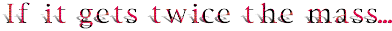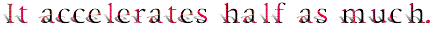A Closer Look At The Proportions

Acceleration is directly proportional to the applied net force.

Below is an animation that illustrates the direct proportion between force and acceleration. There are some notes about this under the animation.

Be sure that you notice:

• The masses, or objects, are the yellow rectangles. All of the masses are the same. So this demonstration does not consider any change in mass. The mass is constant.
• The applied net forces are the red arrows. The forces are not the same. The one at the top is the biggest, the one at the bottom is the smallest, and the one in the middle is medium sized.
• All of the objects accelerate. The velocity in each case gets greater and greater. That is, the speed increases. However, the three accelerations are not all the same. Every one speeds up, but they speed up differently.
• The acceleration at the top is the largest acceleration. The velocity changes by the largest amount per second here. Note that the largest force is applied to this mass. So the largest force has the largest acceleration.
• The acceleration at the bottom is the smallest acceleration. Here the velocity changes by the smallest amount per second. Also, here we have the smallest force. So the smallest applied force creates the smallest acceleration.
• And the medium sized force, in the middle, creates the medium sized acceleration.
• The middle force has one half (1/2) the size of the top force. The middle acceleration has one half (1/2) the size of the top acceleration. You probably can not see this perfectly without taking measurements, but the animation is programed to show it. The acceleration and force change by the same factor, and that is what is meant by a direct proportion between acceleration and force.
• The bottom force has one third (1/3) the size of the top force, and the bottom mass experiences one third (1/3) the acceleration of the top. Both force and acceleration change by the same factor; so, again, it's a direct proportion demonstrated.

Acceleration is inversely proportional to mass.

Following is a program that demonstrates the inverse proportion between acceleration and mass, and then some notes.

What to look for:

• The applied net forces are the red arrows. In each case the force is the same. There is no consideration for changes in force in this demonstration. The applied net force is constant.
• The yellow boxes are the masses. Although all the yellow boxes have the same size, they do not all represent the same mass. The mass is different in each case. Note the labels. The one at the top is the smallest mass, m. The one at the bottom is the largest, 3m, or three times the top mass. The one in the middle is twice as massive as the one at the top.
• All of the objects accelerate. The velocity in each case gets greater and greater. That is, the speed increases. However, the three accelerations are not all the same. All the objects are speeding up; it's the way they are speeding up that is different.
• The acceleration at the top is the largest acceleration. The velocity changes by the greatest amount per second here. Note that here we have the smallest mass. So the smallest mass has the largest acceleration.
• The acceleration at the bottom is the smallest acceleration. Here the velocity changes by the least amount per second. Also, here we have the biggest mass. So the biggest mass has the smallest acceleration.
• And the medium sized mass, in the middle, has the medium sized acceleration.
• The middle mass has twice, or two times, the mass of the top object. The middle acceleration has one half the size of the top acceleration. The demonstration is programed that way, although this may not be apparent without measurement. So the mass changes by a factor of two (2) and the acceleration changes by a factor of one half (1/2). Those factor changes are reciprocals, or inverses, and there is demonstrated an inverse proportion between acceleration and mass.
• At the bottom we have three (3) times the force and one third (1/3) the acceleration. Again, this is an inverse proportion between acceleration and mass, since these factor changes are reciprocals, or inverses.

Newton's Second Law of Motion is often summarized with the equation:

F = ma

This equation is interpreted as:

The applied net force on an object is equal to the mass of the object multiplied by the acceleration of the object.

Click here (or any of the above links) to get to a page that explains this equation and why it is a concise and sufficient statement for Newton's Second Law of Motion.

Click here and link to a collection of other ways to say Newton's Second Law. An explanation for each way is included. Hopefully, the explanation will show that each way of saying this law actually describes the same physics.

Custom Search